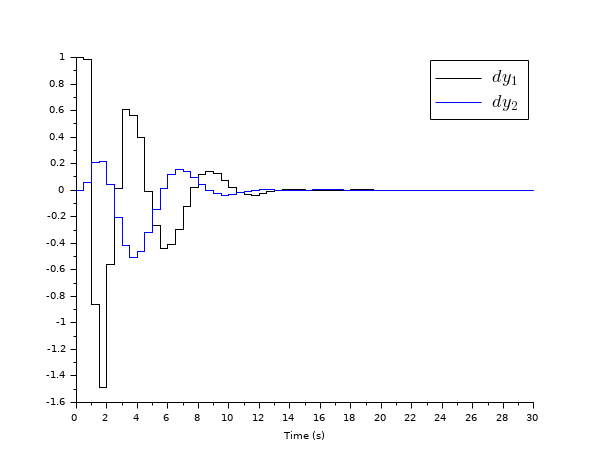Scilab Home page | Wiki | Bug tracker | Forge | Mailing list archives | ATOMS | File exchange
Scilab 6.0.2
Change language to: English - Français - Português - Русский

# lqg

LQG compensator

### Syntax

K = lqg(P_aug, r)
K = lqg(P, Qxu, Qwv)
K = lqg(P, Qxu, Qwv, Qi, #dof)

### Arguments

P_aug

State space representation of the augmented plant (see: lqg2stan)

r

1 by 2 row vector = (number of measurements, number of inputs) (dimension of the 2,2 part of P_aug)

P

State-space representation of the nominal plant (nu inputs, ny outputs, nx states).

Qxu

Symmetric nx+nu by nx+nu weighting matrix.

Qwv

Symmetric nx+ny by nx+ny covariance matrix.

Qi

Symmetric ny by ny weight for integral term.

#dof

Scalar with value in {1,2}, the degrees of freedom of the controller. The default value is 2.

K

Linear LQG (H2) controller in state-space representation.

### Description

#### Regulation around zeroSyntax K=lqg(P_aug,r)

Computes the linear optimal LQG (H2) controller for the "augmented" plant P_aug which can be generated by lqg2stan givent the nominal plant plant P, the weighting matrix Qxu and the noise covariance matrix Qwv.

Syntax K=lqg(P,Qxu,Qwv)

Computes the linear optimal LQG (H2) controller for the nominal plant P, the weighting matrix Qxu and the noise covariance matrix Qwv

#### Regulation around a reference signal, Syntax K=lqg(P,Qxu,Qwv,Qi [,#dof])Computes the linear optimal LQG (H2) reference tracking controller for the plant P, the weighting matrix Qxu and the noise covariance matrix Qwv

### Examples

Assume the dynamical system formed by two masses connected by a spring and a damper:A force(where e is a noise) is applied to M, the deviations dy1 and dy2 from equilibrium positions of the masses are measured. These measures are subject to an additionnal noise v.

A continuous time state space representation of this system is:The instructions below can be used to compute a LQG compensator of the discretized version of this dynamical system. e and v are discrete time white noises such asThe LQ cost is defined by#### Regulation around zero

// Form the state space model
M = 1; m = 0.2; k = 0.1; b = 0.004;
A = [0     1    0    0
-k/M  -b/M  k/M  b/M
0     0    0    1
k/m  b/m  -k/m  -b/m];
B = [0; 1/M; 0; 0];
C = [1 0 0 0  //dy1
0 0 1 0];//dy2
//inputs u and e; outputs dy1 and dy2
P = syslin("c",A, B, C);
// Discretize it
dt=0.5;
Pd=dscr(P, dt);

// The noise variances
R_vv=0.0001*eye(2,2); //measurement noise variance
Q_ww=Pd.B*Q_e*Pd.B'; //input noise adds to regular input u
Qwv=sysdiag(Q_ww,R_vv);
//The compensator weights
Q_xx=diag([0.1 0 5 0]); //Weights on states
R_uu   = 0.3; //Weight on input
Qxu=sysdiag(Q_xx,R_uu);

//----syntax [K,X]=lqg(P,Qxu,Qwv)---
J=lqg(Pd,Qxu,Qwv);

//----syntax [K,X]=lqg(P_aug,r)---
// Form standard LQG model
[Paug,r]=lqg2stan(Pd,Qxu,Qwv); // Form standard LQG model
J1=lqg(Paug,r);

//  Form the closed loop
Sys=Pd/.(-J);
// Compare real and Estimated states for initial state evolution
t = 0:dt:30;
// Simulate system evolution for initial state [1;0;0;0;
y = flts(zeros(t),Sys,eye(8,1));
clf;
plot2d(t',y')
e=gce();e.children.polyline_style=2;
L=legend(["$dy_1$","$dy_2$"]);L.font_size=4;
xlabel('Time (s)')#### Regulation around a reference signal, Syntax K=lqg(P,Qxu,Qwv,Qi [,#dof])

The purpose of the controller is here to assign dy2 using the measure of dy2.

M = 1; m = 0.2; k = 0.1; b = 0.004;
A = [0     1    0    0
-k/M  -b/M  k/M  b/M
0     0    0    1
k/m  b/m  -k/m  -b/m];
B = [0; 1/M; 0; 0];
C = [1 0 0 0  //dy1
0 0 1 0];//dy2
//inputs u and e; outputs dy1 and dy2
P = syslin("c",A, B, C);
// Discretize it
dt=0.1;
Pd=dscr(P, dt);

// The noise variances
R_vv=0.0001; //measurement noise variance
Q_ww=Pd.B*Q_e*Pd.B'; //input noise adds to regular input u
Qwv=sysdiag(Q_ww,R_vv);
//The compensator weights
Q_xx=diag([0.1 0 1 0]); //Weights on states
R_uu   = 0.1; //Weight on input
Qxu=sysdiag(Q_xx,R_uu);

//Control of the second mass position (y2)
Qi=50;
J=lqg(Pd(2,:),Qxu,Qwv,Qi);
H=lft([1;1]*Pd(2,:)*(-J),1);
//step response
t=0:dt:15;
r=ones(t);
dy2=flts(r,H);
clf;
subplot(211);plot(t',dy2');xlabel("Time");ylabel("dy2")
u=flts([r;dy2],J);
subplot(212);plot(t',u');xlabel("Time");ylabel("u")### Reference

Engineering and Scientific Computing with Scilab, Claude Gomez and al.,Springer Science+Business Media, LLC,1999, ISNB:978-1-4612-7204-5

• lqg2stan — LQG を標準問題に変換する
• lqr — LQ compensator (full state)
• lqe — linear quadratic estimator (Kalman Filter)
• h_inf — 連続時間H無限大 (中心) コントローラ
• obscont — オブザーバベース コントローラ

### History

 バージョン 記述 6.0 lqg(P,Qxu,Qwv) and lqg(P,Qxu,Qwv,Qi,#dof) syntaxes added.